# Model accuracy fluctuates after a certain point

Hi.

I’m near done with my classifier. What I’ve noticed is that in my last 2 sets of epochs, the model accuracy starts fluctuating. I have a total of 6 sets of 5 epochs. In the first 4 stages, the model accuracy progresses significantly. In the 5th and the 6th stages, the model just fluctuates around the same value.

This is stage 1. At the beginning of each stage, I get a new learning rate.

``````learn.lr_find()
learn.recorder.plot(suggestion=True)
``````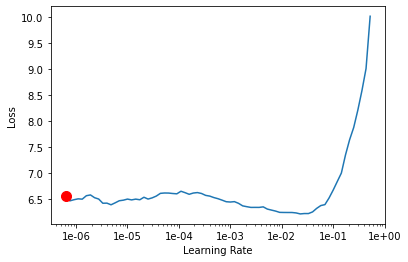``````learn.fit_one_cycle(5, max_lr=slice(1e-061, 1e-04))
learn.save('stage-1')
``````
epoch train_loss valid_loss accuracy top_k_accuracy time
0 4.130252 2.912444 0.399604 0.399604 07:38
1 3.199883 2.535787 0.500726 0.500726 07:43
2 3.067937 2.439397 0.528713 0.528713 07:45
3 2.918948 2.414262 0.535842 0.535842 07:45
4 2.833793 2.400346 0.540264 0.540264 07:46Stage 2

``````learn.unfreeze()
learn.lr_find()
learn.recorder.plot(suggestion=True)
``````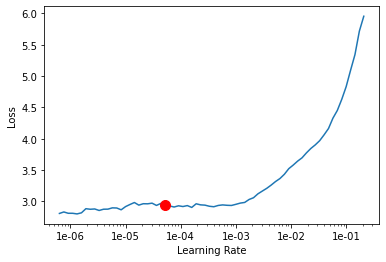``````learn.fit_one_cycle(5, max_lr=slice(1e-05, 1e-04))
learn.save('stage-2')
``````
epoch train_loss valid_loss accuracy top_k_accuracy time
0 2.555121 2.084548 0.639142 0.639142 08:57
1 2.221465 1.954635 0.693069 0.693069 09:03
2 2.031895 1.824991 0.737096 0.737096 09:04
3 1.822814 1.748656 0.767921 0.767921 09:03
4 1.729685 1.727482 0.773795 0.773795 09:02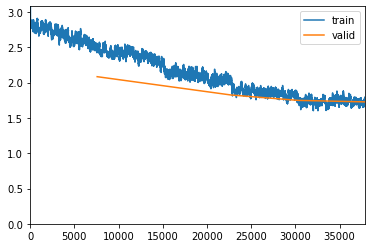Stage 3

``````learn.unfreeze()
learn.lr_find()
learn.recorder.plot(suggestion=True)
``````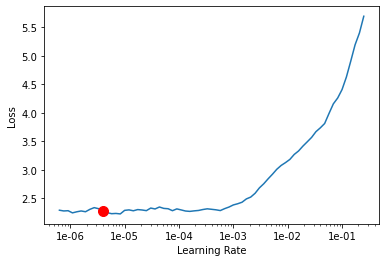``````learn.fit_one_cycle(5, max_lr=slice(1e-06, 1e-04))
learn.save('stage-3')
``````
epoch train_loss valid_loss accuracy top_k_accuracy time
0 1.903848 1.641522 0.795512 0.795512 18:11
1 1.761637 1.599455 0.811881 0.811881 18:12
2 1.740206 1.540293 0.831023 0.831023 18:12
3 1.576381 1.509733 0.835050 0.835050 18:13
4 1.535710 1.500492 0.839076 0.839076 18:12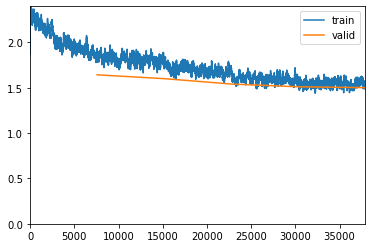Stage 4. Notice the accuracy rate:

``````learn.unfreeze()
learn.lr_find()
learn.recorder.plot(suggestion=True)
``````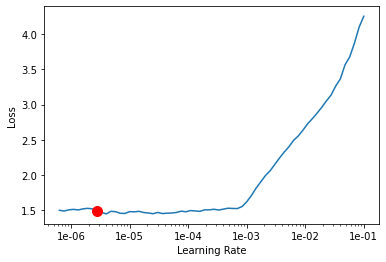``````learn.fit_one_cycle(5, max_lr=slice(1e-06, 1e-04))
learn.save('stage-4')
``````
epoch train_loss valid_loss accuracy top_k_accuracy time
0 1.580565 1.509043 0.835842 0.835842 18:11
1 1.540628 1.514179 0.829769 0.829769 18:12
2 1.436278 1.471246 0.837426 0.837426 18:12
3 1.425504 1.455251 0.841452 0.841452 18:14
4 1.422862 1.447459 0.844158 0.844158 18:13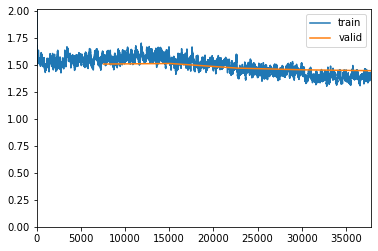Stage 5 isn’t any different so I’ll avoid repeating myself. One detail is that I am increasing the image size to 512 from 224 after stage 3 so I think that might have something to do with it as well. However, when I ran `learn.validate()` by loading stage 3, 4, 5 against my test set, I did not see a decrease in accuracy:

``````path = '/notebooks/food-101/images'

data_test = ImageList.from_folder(path).split_by_folder(train='train', valid='test').label_from_re(file_parse).transform(size=512).databunch().normalize(imagenet_stats)

learn = cnn_learner(data, models.resnet50, metrics=[accuracy, top_1],callback_fns=ShowGraph)